## How to Split Cells by the First Space in Texts in Excel

Sometimes we may use space to separate texts to different groups in one cell, if we want to split this cell to multiple columns refer to space, we can implement this via ‘Text to Columns’ feature. But is there any way to split one cell to only two cells by the first space in the texts? In this free tutorial, we will share you some useful functions in excel to split cells per your demand.

Precondition:

In product list we use space to separate product properties.

Now we want split it to two parts in two different columns by the first space. See expectation below:

## 1. Split Cells to Two Columns by the First Space in Texts by Formula

Step1: In B2 enter the formula:

``=LEFT(A2,FIND(" ",A2)-1)``

Step2: Click Enter to get result. Verify that text before the first space is extracted and saved in B2 properly.

Step3: Drag the fill handle down till the end of the list. Verify that texts from the first space are displayed properly.

Step4: In C2 enter the below formula to extract the left texts after the first space.

``=RIGHT(A2,LEN(A2)-FIND(" ",A2))``

Step5: Click Enter to get result. Verify that texts are displayed properly.

Step6: Drag the fill handle down till the end of the list. Now texts are split to two columns by the first space properly.

## 2. Split Cells to Two Columns by the First Space in Texts with VBA Code

You can use a VBA Code that prompts the user to select a range of cells and a destination cell, and then splits the cells in the selected range to two columns based on the first space in the text.

Step1: Press Alt + F11 to open the Visual Basic Editor.

Step2: In the Visual Basic Editor, go to “Insert” on the top menu and select “Module“.

Step3: Paste the below VBA code into the new module.

```Sub SplitCellsByFirstSpace_ExcelHow()
' Prompt the user to select a range of cells
Dim selectedRange As Range
Set selectedRange = Application.InputBox("Select a range of cells:", Type:=8)

' Prompt the user to select a destination cell
Dim destinationCell As Range
Set destinationCell = Application.InputBox("Select a destination cell:", Type:=8)

' Insert the formula to split the text at the first space
For Each cell In selectedRange
cell.Offset(0, 2).Formula = "=RIGHT(" & cell.Address(False, False) & ",LEN(" & cell.Address(False, False) & ")-FIND("" "", " & cell.Address(False, False) & ",1))"
cell.Offset(0, 1).Formula = "=LEFT(" & cell.Address(False, False) & ",FIND("" "", " & cell.Address(False, False) & ",1)-1)"
Next cell

' Copy the formula to the destination cell and adjacent cell
selectedRange.Offset(0, 1).Resize(selectedRange.Rows.Count, 2).Copy destinationCell

' Convert the formula to values in the destination cells
destinationCell.Resize(selectedRange.Rows.Count, 2).Value = destinationCell.Resize(selectedRange.Rows.Count, 2).Value
End Sub
```

Step4: Press F5 to run the macro or click the “Run” button on the toolbar.

Step5: A dialog box will appear asking you to select a range of cells. Click and drag your mouse to select the range of cells you want to split.

Step6: Another dialog box will appear asking you to select a destination cell. Click on the cell where you want to place the split data.

Step7: The macro will split the data in the selected range into two columns based on the first space in the text, and place the split data into the destination cell and adjacent cells.

## 3. Video: Split Cells to Two Columns by the First Space in Texts

This video will demonstrate how to split cells to two columns by the first space in texts using Excel formulas and VBA code.

## 4. Related Functions

• Excel LEFT function
The Excel LEFT function returns a substring (a specified number of the characters) from a text string, starting from the leftmost character.The LEFT function is a build-in function in Microsoft Excel and it is categorized as a Text Function.The syntax of the LEFT function is as below:= LEFT(text,[num_chars])…t)…
• Excel FIND function
The Excel FIND function returns the position of the first text string (sub string) within another text string.The syntax of the FIND function is as below:= FIND(find_text, within_text,[start_num])…
• Excel RIGHT function
The Excel RIGHT function returns a substring (a specified number of the characters) from a text string, starting from the rightmost character.The syntax of the RIGHT function is as below:= RIGHT (text,[num_chars])…
• Excel LEN function
The Excel LEN function returns the length of a text string (the number of characters in a text string).The syntax of the LEN function is as below:= LEN(text)…

## How to Remove the First/Last Word from Text string in Cell

This post will guide you how to remove the first and the last word from a text string in cells using a formula or User defined function with VBA code in Excel 2013/2016/2019/365. How do I use a formula to remove first and last word of a text string in Excel.

## 1. Remove the First Word from Text String using Formula

If you want to remove the first word from a text string in cells in Excel, you can use a formula based on the RIGHT function, the LEN function and the FIND function. Like this:

``=RIGHT(B1,LEN(B1)-FIND(" ",B1))``

Type this formula into a blank cell and press Enter key on your keyboard and then drag the AutoFill Handle down to other cells to apply this formula.

## 2. Remove the Last Word from Text String using Formula

If you want to remove the last word from a text string, you can use a formula based on the LEFT function, the TRIM function, the FIND function, and the SUBSTITUTE function. Like this:

``=LEFT(TRIM(B1),FIND("~",SUBSTITUTE(B1," ","~",LEN(TRIM(B1))-LEN(SUBSTITUTE(TRIM(B1)," ",""))))-1)``

Type this formula into a blank cell and press Enter key on your keyboard and then drag the AutoFill Handle down to other cells to apply this formula.

## 3. Remove the First Word from Text String using User Defined Function with VBA Code

You can create a User Defined Function in VBA to remove the first word from a text string in Excel by following these steps:

Step1: Open the Visual Basic Editor (VBE) by pressing Alt+F11.

Step2: In the VBE, click on Insert -> Module to create a new module.

Step3: In the module, enter the following code:

```Function RemoveFirstWord_ExcelHow(ByVal inputString As String) As String
Dim firstSpaceIndex As Integer
firstSpaceIndex = InStr(1, inputString, " ")
If firstSpaceIndex > 0 Then
RemoveFirstWord_ExcelHow = Mid(inputString, firstSpaceIndex + 1, Len(inputString))
Else
RemoveFirstWord_ExcelHow = ""
End If

End Function```

Step5: In a blank cell, enter the following formula:

``=RemoveFirstWord(B1)``

Where B1 is the cell that contains the text string you want to remove the first word from.

Step6: Press Enter to display the result.

## 4. Remove the Last Word from Text String using User Defined Function with VBA Code

If you also want to remove the last word from a text string in excel using a User Defined Function with VBA code, and you can refer to the above steps, and just using the following code:

```Function RemoveLastWord_ExcelHow(ByVal inputString As String) As String
Dim lastSpaceIndex As Integer
lastSpaceIndex = InStrRev(inputString, " ")
If lastSpaceIndex > 0 Then
RemoveLastWord_ExcelHow = Left(inputString, lastSpaceIndex - 1)
Else
RemoveLastWord_ExcelHow = ""
End If
End Function```

In Cell E1, type the following formula, press Enter key to apply it:

``=RemoveLastWord_ExcelHow(B1)``

## 5. Video: Remove the First/Last Word from Text string in Cell

This video will demonstrate how to remove the first/last word from a text string using a formula and VBA code.

## 6. Related Functions

• Excel LEFT function
The Excel LEFT function returns a substring (a specified number of the characters) from a text string, starting from the leftmost character.The LEFT function is a build-in function in Microsoft Excel and it is categorized as a Text Function.The syntax of the LEFT function is as below:= LEFT(text,[num_chars])…t)…
• Excel FIND function
The Excel FIND function returns the position of the first text string (sub string) within another text string.The syntax of the FIND function is as below:= FIND(find_text, within_text,[start_num])…
• Excel RIGHT function
The Excel RIGHT function returns a substring (a specified number of the characters) from a text string, starting from the rightmost character.The syntax of the RIGHT function is as below:= RIGHT (text,[num_chars])…
• Excel Substitute function
The Excel SUBSTITUTE function replaces a new text string for an old text string in a text string.The syntax of the SUBSTITUTE function is as below:= SUBSTITUTE  (text, old_text, new_text,[instance_num])….
• Excel LEN function
The Excel LEN function returns the length of a text string (the number of characters in a text string).The syntax of the LEN function is as below:= LEN(text)…
• Excel TRIM function
The Excel TRIM function removes all spaces from text string except for single spaces between words.  You can use the TRIM function to remove extra spaces between words in a string.The syntax of the TRIM function is as below:= TRIM (text)….

## Extract Email Address from Text

This post will guide you how to extract email address from a text string in Excel. How do I use a formula to extract email address in Excel. How to extract email address from text string with VBA Macro in Excel.

Assuming that you have a list of data in range B1:B5 which contain text string and you want to extract all email addresses from those text string. How to achieve it. You can use a formula or VBA Macro to achieve the result. Let’s see the below introduction.

## 1. Extract Email Address from Text with a Formula

To extract email address from text string in cells, you can use a formula based on the TRIM function, the RIGHT function, the SUBSTITUTE function, the LEFT function, the FIND function, the REPT function and the LEN function. Just like this:

``=TRIM(RIGHT(SUBSTITUTE(LEFT(B1,FIND(" ",B1&" ",FIND("@",B1))-1)," ",REPT(" ",LEN(B1))),LEN(B1)))``

Select the adjacent Cell C1, and type this formula, and press Enter key in your keyboard, and then drag the AutoFill Handle over other cells to apply this formula.

## 2. Extract Email Address from Text with User Defined Function

You can also write a User Defined Function with VBA Code to extract email address quickly, just do the following steps:

Step1: open your excel workbook and then click on “Visual Basic” command under DEVELOPER Tab, or just press “ALT+F11” shortcut.

Step2: then the “Visual Basic Editor” window will appear.

Step3: click “Insert” ->”Module” to create a new module.

Step4: paste the below VBA code into the code window. Then clicking “Save” button.

```Function ExtractEmailFromText(s As String) As String
Dim AtTheRateSignSymbol As Long
Dim i As Long
Dim TempStr As String
Const CharList As String = "[A-Za-z0-9._-]"

AtTheRateSignSymbol = InStr(s, "@")
If AtTheRateSignSymbol = 0 Then
ExtractEmailFromText = ""
Else
TempStr = ""
For i = AtTheRateSignSymbol - 1 To 1 Step -1
If Mid(s, i, 1) Like CharList Then
TempStr = Mid(s, i, 1) & TempStr
Else
Exit For
End If
Next i

If TempStr = "" Then Exit Function

TempStr = TempStr & "@"

For i = AtTheRateSignSymbol + 1 To Len(s)
If Mid(s, i, 1) Like CharList Then
TempStr = TempStr & Mid(s, i, 1)
Else
Exit For
End If
Next i
End If

If Right(TempStr, 1) = "." Then TempStr = Left(TempStr, Len(TempStr) - 1)

ExtractEmailFromText = TempStr
End Function

```

Step5: Type the following formula into blank cells and then press Enter key.

``=ExtractEmailFromText(B1)``

Type this formula into a blank cell and then press Enter key in your keyboard.

Step6: lets see the result:

## 3. Video: Extract Email Address from Text in Excel

This video will demonstrate a step-by-step instruction on how to use the formula and VBA code to extract email addresses from a block of text in Excel, making it easy to manage and organize your contact information.

## Excel Array Construction

This article will talk about how to create one-dimensional array or two-dimensional array by using some functions in Excel. When using array formulas in Excel, we often use functions to construct arrays.

## 1. Generate Array with ROW or COLUMN Functions

Array formulas often need to use “natural number” as parameters of the function, such as the second parameter of the LARGE function, OFFSET function in addition to the first parameter. Through the manual way to enter a constant array will be more trouble, and easy to make mistakes. Then we can use the ROW or COLUMN function in EXCEL to generate a sequence, this method is very convenient and fast.

The following formula produces a vertical array of natural numbers from `1` to `10`.

``{=ROW (1:10)}``

The following formula produces a horizontal array of natural numbers from `1 `to `10`.

``{=COLUMN(A:J)}``

## 2. Generating two-dimensional Array from one-dimensional Array

Below we will show you how to construct a new two-dimensional array with two columns of data.

### a. One-dimensional range rearrangement to generate two-dimensional array

If there is a list of students and the name column of that list contains 10 students’ names, we need to randomly place the students’ names in the name column into the cell range of `5 rows and 2 columns` (a new two-dimensional array).

We can use the following steps to randomize the names of students in `column B` to a `5 row 2 column` range, such as the cell range `E1:F5`.

STEP1# Select the cell range `E1:F5`

STEP2# Enter the following array formula in the formula bar

``=INDEX(B2:B11,RIGHT(SMALL(RANDBETWEEN(A2:A11^0,999)/1%+A2:A11,ROW(1:5)*2-{1,0}),2))``

STEP3# Press CTRL + SHIFT +ENTER shortcut keys to convert the above formula into an array formula.

STEP4# You will see that the students’ names have been randomly placed in a two-dimensional range of array.

Let’s see how this array formula works.

=RANDBETWEEN(A2:A11^0,999)

The RANDBETWEEN function is used to generate an array of 10 values, where the elements are random integers between` 1` and `1000`. Since the elements are randomly generated, the size of the array elements is randomly ordered. The array formula generates an array of random integers as follows.

``={484;203;468;525;702;220;13;163;386;54}``

=RANDBETWEEN(A2:A11^0,999)/1%+A2:A11

The random integer array generated above is multiplied by `100 `and then added to the ordinal array of `1` to `10`. This ensures that the last two digits of the array elements are ordinal numbers `1 `to `10`.

={484;203;468;525;702;220;13;163;386;54}/1%+A2:A11

The above array formula returns the following array:

``{48401;20302;46803;52504;70205;22006;1307;16308;38609;5410}``

=ROW(1:5)*2-{1,0}

The ROW function generates a vertical array` {1;2;3;4;5)`, and then subtracts a constant array {1,0} to produce a two-dimensional array of 5 rows and 2 columns.

=SMALL()

The result is taken as the second argument to the SMALL function, which sorts the array after multiplication and addition processing. Since the original size of the array is random, after sorting, the ordinal number corresponding to the last two digits of each element is randomly ordered.

=RIGHT()

The RIGHT function is used to extract the last two digits of each element, and the INDEX function is used to return the student’s name in the corresponding position in `column B`. In this way, the names in `column B `can be randomly populated into a two-dimensional array of `5 rows and 2 columns`.

### b. Combining two columns of data to create a two-dimensional array

We can use the VLOOKUP function to query from the right to the left, and we can use the array operation and IF function to swap two columns of data to generate a new two-dimensional array.

Here is an example of how the VLOOKUP function can be used to reverse the query by constructing a new array.

If you have a table of employee information, and you need to find the employee’s number by the employee’s name, you can use the VLOOKUP function in combination with the IF function to construct a two-dimensional array to find the corresponding employee number.

The employee information table is as follows:

To find the employee number by name, the steps are as follows.

STEP1# Select the cell `B3`

STEP2# Enter the following formula in the formula bar and press Enter

``=VLOOKUP(B2,IF({1,0},E2:E11,D2:D11),2,)``

STEP3# As you can see, Jerry’s employee number has been found.

Let’s see how the above formula works.

The core part of the formula is `IF ({1,0},E2:E11,D2:D11)`, which uses a horizontal array` {1,0}` and two vertical arrays to perform operations to achieve the position of the column where the employee’s name and work number are swapped. Its returned memory arrays are：

``{"Tom",1;"Jerry",2;"Jacey",3;"Wendy",4;"William",5;"Ocean",6;"scott",7;"charies",8;"ceila",9;"nicole",10}``

The VLOOKUP function then queries the employee’s name in the two-dimensional array generated by the IF function and returns the corresponding employee number.

## 3. Extracting Sub-arrays From Data

In daily work, it is often necessary to extract part of the data from a column and reprocess it. For example, If you want to find out the list of employees who meet the specified requirements in the employee table.

The following describes how to extract some data from a column to form a subarray.

Suppose you have an employee salary table and you want to find out the names of employees whose salary is greater than `\$2000`. The salary table is as follows.

You can refer to the following steps to obtain a list of employees who meet the requirements.

STEP1# First you need to select the cell range` E2:E11`

STEP2# Enter the following formula in the formula bar

``=T(OFFSET(B1,SMALL(IF(C2:C11>2000,A2:A11),ROW(INDIRECT("1:"&COUNTIF(C2:C11,">2000")))),))``

STEP3# Press CTRL + SHIFT +ENTER shortcut keys to convert the above formula into an array formula.

Let’s see how the above formula works.

=IF(C2:C11>2000,A2:A11)

First use the IF function to determine whether the salary meets the conditions, if the salary is greater than \$2000, then return the employee’s ID, otherwise return the logical value FALSE.

=ROW(INDIRECT(“1:”&COUNTIF(C2:C11,”>2000″)))

The COUNTIF function is used to calculate the number of scores greater than `100 `and is combined with the ROW function and INDIRECT function to generate a sequence of natural numbers from `1` to `n`.

=SMALL(IF(C2:C11>2000,A2:A11),ROW( INDIRECT(“1:”&COUNTIF(C2:C11,”>2000″))))

Use the SMALL function to find the employee number whose salary is greater than `\$2000` and return the following memory array.

``={1;3;5;10}``

=OFFSET(B1,{1;3;5;10},)

The OFFSET function extracts the employee’s name from the result returned by the SMALL function and returns the following array of employee names.

``={"Tom";"Jacey";"William";"nicole"}``

=T(OFFSET(B1,{1;3;5;10},))

Finally, the T function is used to convert the multi-dimensional reference returned by the OFFSET function into a memory array.

## 4. Extracting Sub-array from a two-dimensional Array

The cell range `A1:C10` contains data of text and numeric type, see the figure below.

If you want to extract all the text-based data from the specified cell range `A1:C10`, then you can use the following array formula.

``=T(INDIRECT(TEXT(SMALL(IF(A1:C10>="",ROW(A1:C10)/1%+COLUMN( A1:C10)),ROW(INDIRECT("1:"&COUNTIF(A1:C10,"*")))), "r0c00"),))``

Let’s see how the above formula works.

=IF(A1:C10>=””,ROW(A1:C10)/1%+COLUMN( A1:C10))

The IF function is used to determine the type of data in the cell range. If the cell value is the text, then let the cell’s line number multiplied by `100`, and then add the cell’s column number, and then return a numeric result; if the cell value is not text type, then return the logical value `FALSE`.

``={FALSE,102,FALSE;FALSE,202,FALSE;FALSE,302,FALSE;FALSE,402,FALSE;FALSE,502,FALSE;FALSE,602,FALSE;FALSE,702,FALSE;FALSE,802,FALSE;FALSE,902,FALSE;FALSE,1002,FALSE}``

=ROW(INDIRECT(“1:”&COUNTIF(A1:C10,”*”)))

The COUNTIF function is used to calculate the number of text values in the range of cells `A1:C10`, and combined with the ROW function and INDIRECT function to generate a series of natural numbers from 1 to n.

``={1;2;3;4;5;6;7;8;9;10}``

=SMALL(IF(A1:C10>=””,ROW(A1:C10)/1%+COLUMN( A1:C10)),ROW(INDIRECT(“1:”&COUNTIF(A1:C10,”*”))))

The SMALL function is used to extract the position information of the cell where the text is located and return a memory array.

``={102;202;302;402;502;602;702;802;902;1002}``

=INDIRECT(TEXT({102;202;302;402;502;602;702;802;902;1002}, “r0c00”),)

The TEXT function is used to convert the location information to R1C1 reference style, and then use the INDIRECT function to return to the cell reference.

=T(INDIRECT(TEXT({102;202;302;402;502;602;702;802;902;1002}, “r0c00”),))

Finally, the multi-dimensional references returned by the INDIRECT function are converted to memory arrays using the T function.

## 5. Fill the Merged Cells by Array Formula

In the merged cells, only the first cell has a value, while the rest of the cells are empty cells. When we work with the data, we may need to fill the empty cells in the merged cells with the corresponding values to meet the needs of the calculation.

The following is a product sales table, we need to fill the empty cells in the merged cells with the corresponding `region name`. The data table is as follows:

You can fill the data into the merged cells by using the following array formula.

``=LOOKUP(ROW(A2:A13),ROW(A2:A13)/(A2:A13>""),A2:A13)``

Let’s See How This Formula Works:

=ROW(A2:A13)/(A2:A13>””)

This formula assigns a non-empty cell in column A to the row number of that cell, and returns the error value `#DIV/O! `for empty cells, and finally returns a memory array.

``{2;#DIV/0!;#DIV/0!;#DIV/0!;6;#DIV/0!;#DIV/0!;#DIV/0!;10;#DIV/0!;#DIV/0!;#DIV/0!}``

Finally, the LOOKUP function is used to perform a fuzzy search and return the corresponding region name.

## 6. Convert Two-dimensional Array to one-dimensional Array

Some functions only support one-dimensional array as their arguments, not two-dimensional array. For example, the second argument of the MATCH function, the second argument of the LOOKUP function, and so on. If you want to complete the query in a two-dimensional array, you need to first convert the two-dimensional array to a one-dimensional array.

In the figure below, the cell range `A1:C4` is a two-dimensional array, by using the following formula you can return the maximum value less than or equal to `100 `in the cell range; LOOKUP function will perform a fuzzy search from a one-dimensional array returned by the SMALL formula, and return the value that matches the conditions.

The formula is as follows.

``=LOOKUP(100,SMALL(A1:C4,ROW(1:12)))``

Let’s see how this formula works:

=SMALL(A1:C4,ROW(1:12))

Because the cell range is `4 rows and 3 columns`, it is a two-dimensional array containing `12 `elements. You can generate a sequence of natural numbers from `1` to `12 `by using the ROW function. Then use the SMALL function to sort the two-dimensional array and return a one-dimensional memory array. The result is as follows:

``={16;60;78.6;79;97;97;99;#NUM!;#NUM!;#NUM!;#NUM!;#NUM!}``

The LOOKUP function performs a fuzzy lookup by row and ignores the error value `#NUM!`. Finally, the maximum value less than or equal to `100 `is returned, which is `99`.

## How to Convert Military Time to Standard Time in Excel

This post will guide you how to convert military time to standard time with a formula in Excel. How do I convert from military time 17:29:30 to regular time 5:29:30PM in Excel.  How do I Convert Standard time to military time with a formula in Excel.

## 1. Convert Military Time to Standard Time using Formula

Assuming that you have a list of data in range B1:B4, in which contain military time. And you wish to convert those times to standard time. How to do it.

You can a formula based on the TIMEVALUE function, the LEFT function, the MID function and the RIGHT function. The formula is similar to the below:

``=TIMEVALUE(LEFT(B1,2)&":"&MID(B1,3,2)&":"&RIGHT(B1,2))``

Step1: You need to type this formula into a blank cell (at this example, we select Cell C1) and press Enter key, then copy this formula from cell C1 to range C2:C4.

Step2: Then you need to select range C1:C4, and right click on it, select Format Cells from the popup menu list. the Format Cells dialog will open.

Step3: Switch to Number tab in the Format Cells dialog box, and click Time from the Category list box, then select the standard time type in the Type list box. Click Ok button.

Step4: Let’s see the last result:

## 2. Convert Military Time to Standard Time using User Defined Function with VBA Code

If you want to convert Military Time to Standard Time using a User-Defined Function with VBA code, you can follow these steps:

Step1: Press ALT + F11 to open the Visual Basic Editor.

Step2: In the Visual Basic Editor, click on Insert -> Module to insert a new module.

Step3: n the new module, paste the following code. Save the module and return to the Excel workbook.

```Function ConvertMilitaryToStandard_ExcelHow(MilitaryTime As String) As String
Dim Hour As Integer
Dim Minute As String
Dim Second As String
Dim StandardTime As String

Hour = Left(MilitaryTime, 2)
Minute = Mid(MilitaryTime, 3, 2)
Second = Right(MilitaryTime, 2)

If Hour = 0 Then
StandardTime = "12:" & Minute & ":" & Second & " AM"
ElseIf Hour < 12 Then
StandardTime = Hour & ":" & Minute & ":" & Second & " AM"
ElseIf Hour = 12 Then
StandardTime = "12:" & Minute & ":" & Second & " PM"
Else
StandardTime = Hour - 12 & ":" & Minute & ":" & Second & " PM"
End If

ConvertMilitaryToStandard_ExcelHow = StandardTime
End Function```

Step4: In a blank cell, enter the following formula:

``=ConvertMilitaryToStandard_ExcelHow(B1)``

Where B1 is the cell containing the Military Time.

Step5: Press Enter to see the converted time in Standard Time format.

The above VBA code converts the Military Time to Standard Time by extracting the hour and minute components of the Military Time and then using an If-Else statement to determine the corresponding Standard Time.

## 3. Convert Standard Time to Military Time Using Text Formula

If you want to convert Standard time to Military time, you can use another formula based on the TEXT function. Like this:

``=TEXT(C1,"HHMMSS")``

Type this formula into cell D1, press Enter key on your keyboard. Drag the AutoFill Handle over to other cells to apply this formula.

## 4. Video: Convert Military Time to Standard Time in Excel

This video will show you how to convert military time to standard time in Excel using both a formula and VBA code.

## 5. Related Functions

• Excel TIMEVALUE Function
The Excel TIMEVALUE function returns the decimal number of the time represented by a text string. so it will convert a time represented by a text string into an Excel time value.The syntax of the TIMEVALUE function is as below:=TIMEVALUE (time_text)…
• Excel MID function
The Excel MID function returns a substring from a text string at the position that you specify.The syntax of the MID function is as below:= MID (text, start_num, num_chars)…
• Excel LEFT function
The Excel LEFT function returns a substring (a specified number of the characters) from a text string, starting from the leftmost character.The LEFT function is a build-in function in Microsoft Excel and it is categorized as a Text Function.The syntax of the LEFT function is as below:= LEFT(text,[num_chars])…
• Excel RIGHT function
The Excel RIGHT function returns a substring (a specified number of the characters) from a text string, starting from the rightmost character.The syntax of the RIGHT function is as below:= RIGHT (text,[num_chars])…
• Excel Text function
The Excel TEXT function converts a numeric value into text string with a specified format. The TEXT function is a build-in function in Microsoft Excel and it is categorized as a Text Function. The syntax of the TEXT function is as below: = TEXT (value, Format code)…

## How to Remove Last or Trailing Commas in Excel

This post will guide you how to remove trailing commas from cell in Excel. How do I remove comma character at the end of a cell with a formula in Excel 2013/2016.

## 1. Remove Trailing Commas using Formula

if you want to remove trailing commas from you Excel cells, and you can create a formula to check for a comma at the end of a string and then removes the final comma character. you can use the IF function, the RIGHT function, the LEFT function and the LEN function to remove the commas from each cell, like this:

``=IF(RIGHT(A1,1)=",",LEFT(A1,LEN(A1)-1),A1)``

You need to type this formula into a blank cell and press Enter key on your keyboard, and then drag the AutoFill handle over to the range of cells that you want to apply this formula.

## 2. Remove Last or Trailing Commas using User Defined Function with VBA Code

You can also use a user defined function with VBA code to remove last or trailing commas in Excel. Here are the steps to create and use such a function:

Step1: Press Alt + F11 to open the Visual Basic Editor.

Step2: In the Visual Basic Editor window, right-click on any sheet name and select Insert > Module.

Step3: In the code window, paste the following VBA code. Press Ctrl + S to save the workbook as a macro-enabled file (.xlsm).

```Function RemoveLastComma_Excelhow(rng As Range) As String
Dim s As String
s = rng.Value
If Right(s, 1) = "," Then
s = Left(s, Len(s) - 1)
End If
RemoveLastComma_Excelhow = s
End Function
```

Step4: Go back to the worksheet where you have the data with commas.

Step5: In an empty cell, enter the formula:

``=RemoveLastComma_Excelhow(A1)``

Where A1 is the cell that contains the comma-separated values.

Step6: press Enter key and you will see that the last or trailing comma is removed from each cell.

## 3. Video: Remove Last or Trailing Commas

In this video, you will learn how to use the LEFT and LEN functions to extract the text before the last comma, and how to create a User Defined Function with VBA code that can remove the trailing commas.

## 4. Related Functions

• Excel IF function
The Excel IF function perform a logical test to return one value if the condition is TRUE and return another value if the condition is FALSE. The IF function is a build-in function in Microsoft Excel and it is categorized as a Logical Function.The syntax of the IF function is as below:= IF (condition, [true_value], [false_value])….
• Excel LEFT function
The Excel LEFT function returns a substring (a specified number of the characters) from a text string, starting from the leftmost character.The LEFT function is a build-in function in Microsoft Excel and it is categorized as a Text Function.The syntax of the LEFT function is as below:= LEFT(text,[num_chars])…t)…
• Excel RIGHT function
The Excel RIGHT function returns a substring (a specified number of the characters) from a text string, starting from the rightmost character.The syntax of the RIGHT function is as below:= RIGHT (text,[num_chars])…
• Excel LEN function
The Excel LEN function returns the length of a text string (the number of characters in a text string).The syntax of the LEN function is as below:= LEN(text)…

## Sort by Second or Third character in a Column

This post will guide you how to sort text string by second or third character in a Column in Excel. You may be want to sort a string in a Cell by the Second or last character in excel, how to quickly do it? You can use the Excel Formula or Text to Column feature to solve this problem. The below will give you the detailed description.

## 1. Video: Sort by Second Character in a Column

In the below video tutorial, you can learn how to sort by second or third character in a column by using a formula with the RIGHT function or by splitting the text into columns with the Text to Column feature.

## 2. Excel sort by second character with Formula

To sort test string in a cell by its second character, you can use a combination of the RIGHT function and the LEN function to build a new Excel formula, you can refer to the following generic formula.

``=RIGHT(Cell, LEN(Cell)-1)``

This formula will remove the first character of the text string in Cell, then you can use the sort feature in Microsoft Excel to sort this column, and then the original column also will be sorted. Just do it following:

Assuming that you want to sort the range A1:A4 in Column A by second character.

Step1: select the cell B1 next to the cell A1 contains that you want to sort

Step2: type the following formula in the formula box of Cell B1, then press Enter key.

``=RIGHT(A1,LEN(A1)-1)``
`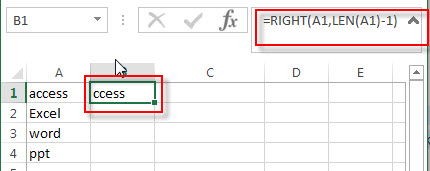`

Step3: select Cell B1, then drag the AutoFill Handle down to the cell B2:B4 to apply this formula. You will see that the first character will be deleted.

Step4: select the range B1:B4, on the DATA tab, click sort A to Z command under Sort & Filter group.

Step5: choose Expand the selection radio button in the Sort Warning window. Then click Sort button.

Step6: you will see that the column A and B are sorted by the second character.

Step7: you can remove the column B now.

## 3. Excel sort by second character with Text to Columns

If the text strings are joined by the delimited character, and you want sort text string by the middle characters, then you can use the Text to Columns feature to achieve the result. Or you can use the MID function to extract the middle characters, then use the Sort feature in Excel to sort them.

Just refer to the following steps:

Step1: select the range of cells contain the text string that you want to sort.

Step2: on the DATA tab, click Text to Column command, the Convert Text to Columns Wizard window will appear.

Step3: choose Delimited radio button in the first step window, click Next button

Step4: type the delimited character which is used to join the text string in Cell, click Next button

Step5: choose the destination cell reference, then click Finish button.

Step6: you will see that the text string is split into three columns, and then you can select the column that contain the middle characters, click Sort A to Z command under DATA tab, the columns are sorted by the middle characters in each cell.

This method is only available for the text string that joined by the specific character or delimiter. So if the text string in Cell do not have any common delimiter, you can use the MID function to extract the middle characters, then following the Step5 to sort the text string. You can use the following generic formula:

``=MID(A1,FIND("-",A1)+1,1)``

## 4. Related Formulas

• Sort Names by Middle Name in Excel
Assuming that you have a list of names in your worksheet and you would like to alphabetize by middle name. You can create an excel formula based on the IF function, the ISERR function, the FIND function, and the MID function.…
• Split Multiple Lines from a Cell into Rows
If you have multiple lines in a cell and each line is separated by line break character or press “alt + enter” key while you entering the text string into cells, and you need to extract the multiple lines into the separated rows or columns, you can use a combination with the TRIM function, the MID function, the SUBSTITUTE function, the REPT function, the LEN function to create a complex excel formula..…
• Extract nth word from text string
If you want to extract the nth word from a text string in a single cell, you can create an excel formula based on the TRIM function, the MID function, the SUBSTITUTE function, the REPT function and the LEN function..…
• Get last word from text string
If you want to get the last word from a text string, you can create an excel formula based on the RIGHT function, the LEN function, the FIND function and the SUBSTITUTE function..…
• Extract word that starting with a specific character
Assuming that you have a text string that contains email address in Cell B1, and if you want to extract word that begins with a specific character “@” sign, you can use a combination with the TRIM function, the LEFT function, the SUBSTITUTE function, the MID function, the FIND function, the LEN function and the REPT function to create an excel formula.…

## 5. Related Functions

• Excel RIGHT function
The Excel RIGHT function returns a substring (a specified number of the characters) from a text string, starting from the rightmost character.The syntax of the RIGHT function is as below:= RIGHT (text,[num_chars])…
• Excel LEN function
The Excel LEN function returns the length of a text string (the number of characters in a text string).The syntax of the LEN function is as below:= LEN(text)…
• Excel FIND function
The Excel FIND function returns the position of the first text string (sub string) within another text string.The syntax of the FIND function is as below:= FIND(find_text, within_text,[start_num])…
• Excel MID function
The Excel MID function returns a substring from a text string at the position that you specify.The syntax of the MID function is as below:= MID (text, start_num, num_chars)….

## Extract substring In Excel

This post will guide you how to use Excel’s MID function is a quick and easy way to extract pieces from your text. Use the Excel formula to extract a substring with MID.

Note: If you want to extract just a single character at any given time, use the “start” and end positions as your literal start/endpoints. However, if you know how many characters there will be in total (e.g., less than 10), then instead of plugging those numbers into our formula directly. They can easily become calculated values by dividing each side differently!

In the above example, we are going to extract text from a range of cells based on certain criteria. The arguments of our three-argument functions include

1) A text string

2) Starting cell number in B2 with linefeeds replaced by spaces (to make it easier for you).

3) The amount that needs to be removed before reaching C2, where we’ll find out how many characters were actually enclosed between those two points inclusive.

Each time round, these calculations happen, subtracting one point until there’s nothing left but space or a negative #.

Note: Ensure that you have the Text to Columns feature enabled in Excel before attempting this formula; otherwise, it won’t work!

=MID(A2,B2,C2-B2+1)

=MID(“free excel tutorial excelhow “,1,4-1+1)

=MID(A2,1,4)

## Extract Substrings Functions

There are three main extracting substrings functions that are Excel provides.

=MID(Text,start,chars) // extract from middle

=LEFT(Text,chars) // extract from left

=RIGHT(Text,chars) // extract from right

With This Formula Find Start And End Positions:

To handle what start and end positions are, consider the following example.

=MID(B5&C5&D5,”red”,2) // extracts “ed”

The start position of 2 is after the 3rd character, i.e., “r”. Therefore, the number of characters you wish to extract must begin from the start position.

There is no use of Start and end points in the formula because we already know how many characters we want to extract.

### Related Functions

• Excel MID function
The Excel MID function returns a substring from a text string at the position that you specify.The syntax of the MID function is as below:= MID (text, start_num, num_chars)…
• Excel LEFT function
The Excel LEFT function returns a substring (a specified number of the characters) from a text string, starting from the leftmost character.The LEFT function is a build-in function in Microsoft Excel and it is categorized as a Text Function.The syntax of the LEFT function is as below:= LEFT(text,[num_chars])…
• Excel RIGHT function
The Excel RIGHT function returns a substring (a specified number of the characters) from a text string, starting from the rightmost character.The syntax of the RIGHT function is as below:= RIGHT (text,[num_chars])…

## How to Convert Uppercase to Lowercase Except the First Letter in Excel

In excel, words can be entered in uppercase, lowercase or mixed. If we want to convert all uppercase to lowercase (except the first letter in some situations), we can use formula with some letter convert related functions to convert them to proper case. This article will introduce you some methods to convert letters from uppercase to lowercase, it can help you to solve your problem.

Precondition:

Prepare below table. To convert uppercase to lowercase for worlds or sentence, actually there are two forms after converting. We can convert uppercase to lowercase but keep the first uppercase for each word; in another way, we can convert uppercase to lowercase but only keep the first uppercase for the first word in the sentence.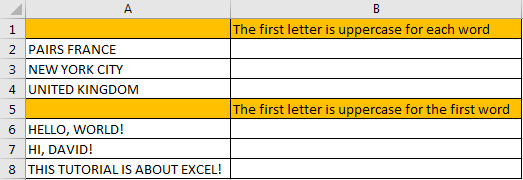## Part 1: Convert Uppercase to Lowercase Except the First Letter for Each Word

Step 1: In B2 enter the formula =PROPER(A2).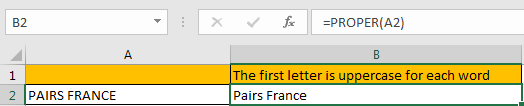For PROPER function, it converts a text or string to proper case, actually it converts the first letter in each word to uppercase, but keeps other letters in lowercase. So, no matter letters are entered in uppercase or lowercase or mixed, it will be finally displayed with the first letter in uppercase with the other letters in lowercase after applying this function.

Step 2: Drag the fill handle down to fill other cells. Verify that uppercase is converted to lowercase properly.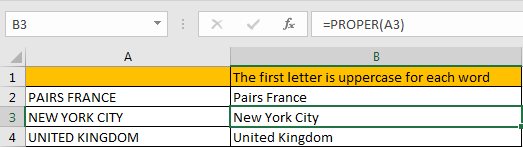## Part 2: Convert Uppercase to Lowercase Except the First Letter for the First Word

Step 1: In B6 enter the formula =UPPER(LEFT(A6,1))&LOWER(RIGHT(A6,LEN(A6)-1)).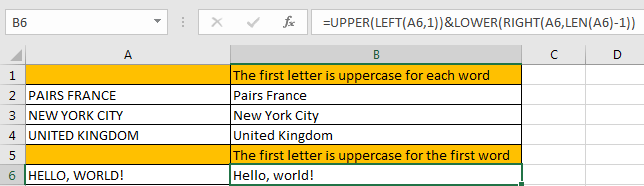This formula is combined with UPPER and LOWER two functions. It is easy to understand. For UPPER function, keep the first letter from left displaying in uppercase; for LOWER function, keep other letters except the first letter from left displaying in lowercase.

Step 2: Drag the fill handle down to fill other cells. Verify that uppercase is converted to lowercase properly.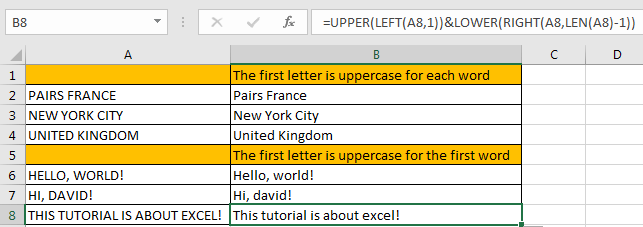### Related Functions

• Excel UPPER function
The Excel UPPER function converts all characters in text string to uppercase.The UPPER function is a build-in function in Microsoft Excel and it is categorized as a Text Function.The syntax of the UPPER function is as below:= UPPER (text)…
• Excel LOWER function
The Excel LOWER function converts all characters in a text string to lowercase.The syntax of the LOWER function is as below:= LOWER  (text)…
• Excel LEFT function
The Excel LEFT function returns a substring (a specified number of the characters) from a text string, starting from the leftmost character.The LEFT function is a build-in function in Microsoft Excel and it is categorized as a Text Function.The syntax of the LEFT function is as below:= LEFT(text,[num_chars])…
• Excel RIGHT function
The Excel RIGHT function returns a substring (a specified number of the characters) from a text string, starting from the rightmost character.The syntax of the RIGHT function is as below:= RIGHT (text,[num_chars])…
• Excel LEN function
The Excel LEN function returns the length of a text string (the number of characters in a text string).The syntax of the LEN function is as below:= LEN(text)…

## How to Convert Text to Time in Excel

This post will guide you how to convert time string to time in Excel. Assuming that you have a list of text string in your worksheet, and you wish to convert these time string to standard time format, how to do it.

You need to use an formula based on the TIMEVALUE function, the LEFT function, the LEN function and the RIGHT function. Like below:

`=TIMEVALUE(LEFT(B1,LEN(B1)-2)&":"&RIGHT(B1,2))`

Type this formula into a blank cell, and press Enter key on your keyboard to apply it. and drag the AutoFill Handle to other cells to apply this formula.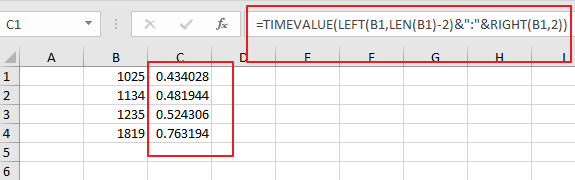Then you need to keep these cells selected, and right click on it, select Format Cells from the context menu list and the Format Cell Dialog will open.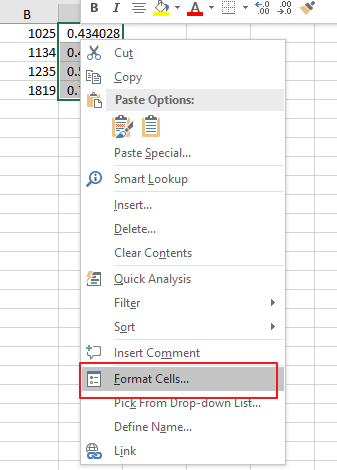Switch to Number tab under Format Cells dialog box, and select Time category from the Category list, and select one time type as you need. click Ok button.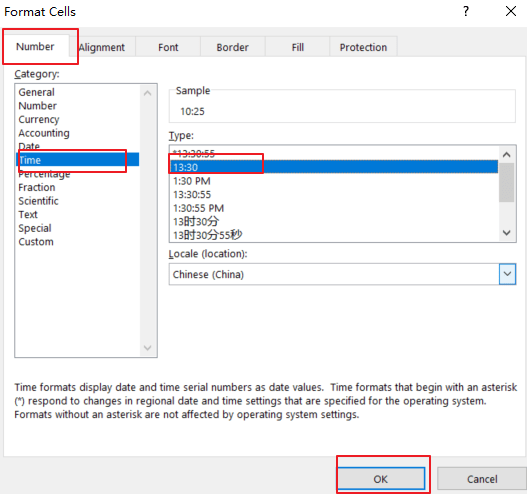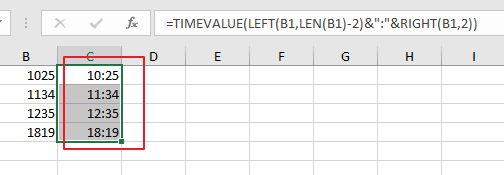If you want to convert date and time string to a data time format in Excel, and you can use the following formula based on the DATE function, the LEFT function, the MID function, the Time Function and the RIGHT function. Like below:

`=DATE(LEFT(B1,4),MID(B1,5,2),MID(B1,7,2))+TIME(MID(B1,10,2),RIGHT(B1,2),0)`

Type this formula into a blank cell and press Enter key on your keyaboard.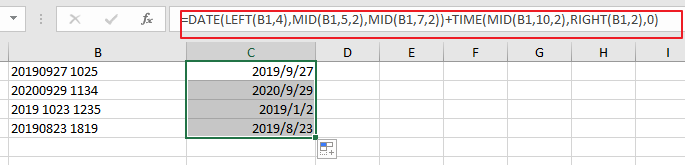Then you need to format those selected cells to a data format as you need.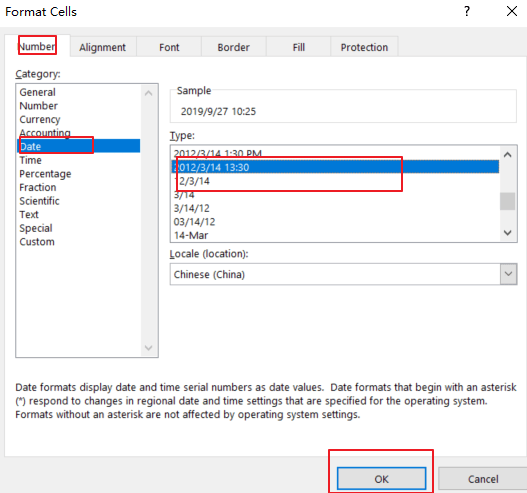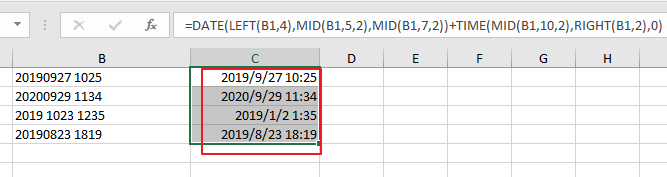### Related Functions

• Excel TIMEVALUE Function
The Excel TIMEVALUE function returns the decimal number of the time represented by a text string. so it will convert a time represented by a text string into an Excel time value.The syntax of the TIMEVALUE function is as below:=TIMEVALUE (time_text)…
• Excel MID function
The Excel MID function returns a substring from a text string at the position that you specify.The syntax of the MID function is as below:= MID (text, start_num, num_chars)…
• Excel LEFT function
The Excel LEFT function returns a substring (a specified number of the characters) from a text string, starting from the leftmost character.The LEFT function is a build-in function in Microsoft Excel and it is categorized as a Text Function.The syntax of the LEFT function is as below:= LEFT(text,[num_chars])…
• Excel RIGHT function
The Excel RIGHT function returns a substring (a specified number of the characters) from a text string, starting from the rightmost character.The syntax of the RIGHT function is as below:= RIGHT (text,[num_chars])…
• Excel TIME function
The Excel TIME function returns a date in serial number format for a particular time (the hour, minute and second).The syntax of the TIME function is as below:= TIME(hour, minute, second)…

## How to Convert mmddyy to Date in Excel

This post will guide you how to convert non-standard date formats or text to a standard date in Excel. If you have a date with mmddyy format, and you want to change it as the normal date format as mm/dd/yyyy with Excel Formula. How do I convert the mmddyyyy text to normal date format in Excel 2013/2014.

Assuming that you have a list of data in range B1:B4 which contain date values as text format. and you can use one of the following methods to convert it as normal date format.

## Convert mmddyyyy Text to Date with Formula

To convert the mmddyyyy text to normal date format, you can use a formula based on the DATE function, the MID function and the RIGHT function. Just like this:

`=DATE(RIGHT(B1,4),LEFT(B1,2),MID(B1,3,2))`

Type this formula into a blank cell and press Enter key, and then drag the AutoFill Handle down to other cells to apply this formula to convert it.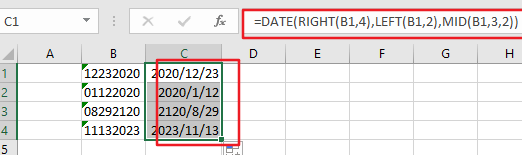## Convert mmddyy Text to Date with Text To Column Feature

You can also convert mmddyyyy Text to a normal date format with Text to Column feature in Excel. Just do the following steps:

Step1:select the range of cells that you want to convert, such as: B1:B4.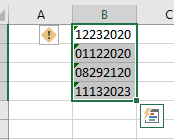Step2: go to Data tab in the Excel Ribbon, and click Text to Column command under Data Tools group. And the Convert Text to Columns dialog box will appear.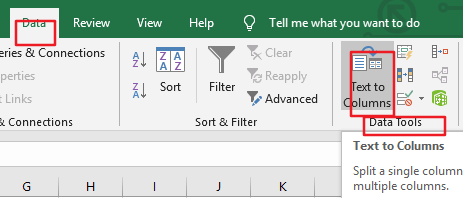Step3: select Delimited option under Original Data type section in the Convert Text to Columns dialog box, and click Next button. Click Next button again.

Step4: select Date option under the Column Data format, and then choose MDY from the drop down list box of Date. Then select on destination cell to place data. Click Finish button.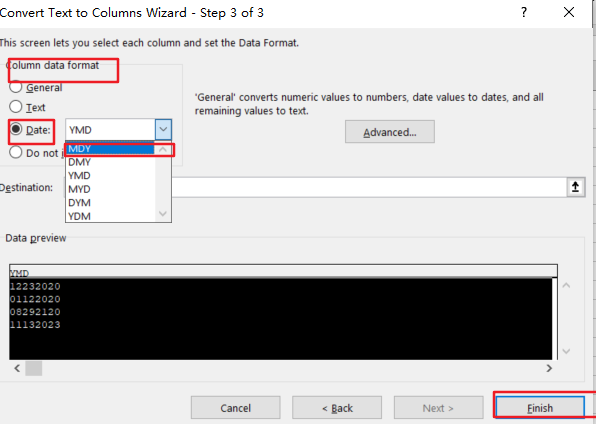Step5: you would see that the selected cells have been converted to a normal date format.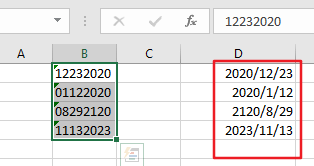## Convert mmddyy Text to Date with VBA Macro

If you want to convert mmddyyyy text to a normal date format quickly, you can also use an Excel VBA Macro to achieve the same result. Just do the following steps:

Step1: open your excel workbook and then click on “Visual Basic” command under DEVELOPER Tab, or just press “ALT+F11” shortcut.Step2: then the “Visual Basic Editor” window will appear.

Step3: click “Insert” ->”Module” to create a new module.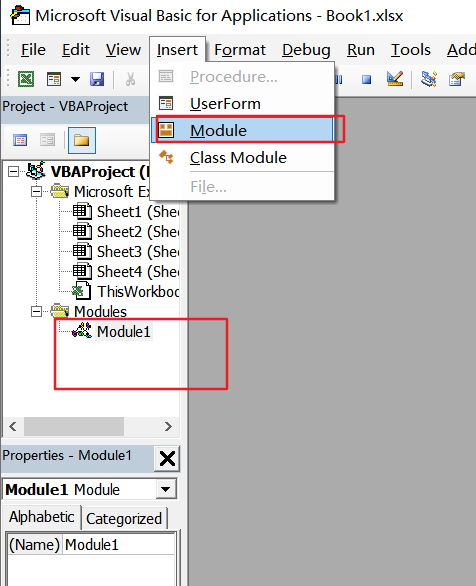el v

Step4: paste the below VBA code  into the code window. Then clicking “Save” button.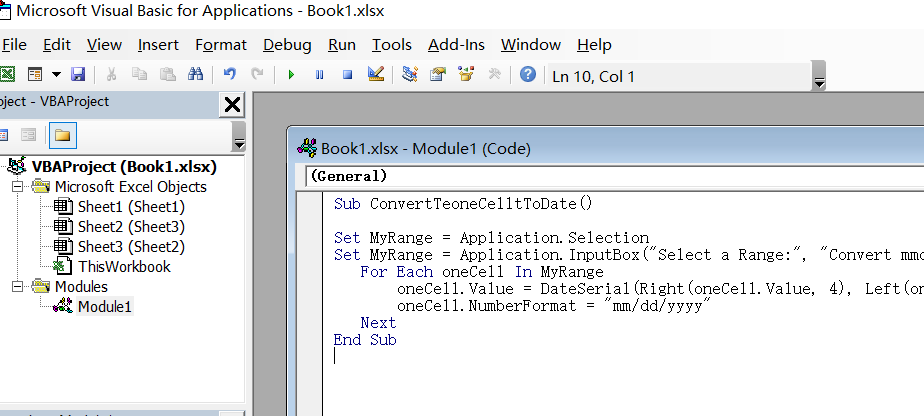```Sub ConvertTeoneCelltToDate()
Set MyRange = Application.Selection
Set MyRange = Application.InputBox("Select a Range:", "Convert mmddyyy to date", MyRange.Address, Type:=8)
For Each oneCell In MyRange
oneCell.Value = DateSerial(Right(oneCell.Value, 4), Left(oneCell.Value, 2), Mid(oneCell.Value, 3, 2))
oneCell.NumberFormat = "mm/dd/yyyy"
Next
End Sub```

Step5: back to the current worksheet, then run the above excel macro. Click Run button.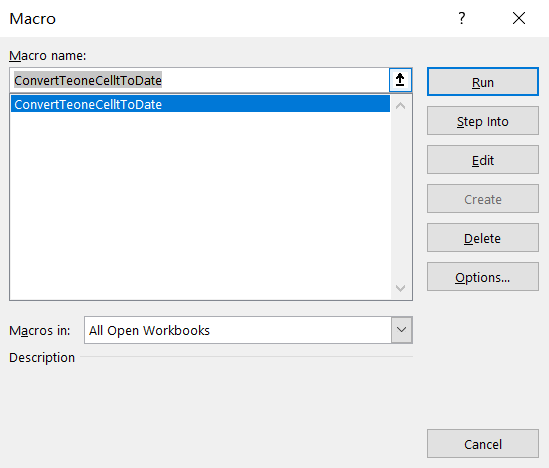Step6: select one range which contain text data that you want to convert, such as: B1:B4. Click OK button.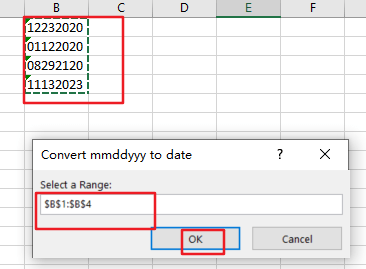Step7: Let’s see the result: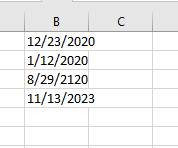### Related Functions

• Excel MID function
The Excel MID function returns a substring from a text string at the position that you specify.The syntax of the MID function is as below:= MID (text, start_num, num_chars)…
• Excel LEFT function
The Excel LEFT function returns a substring (a specified number of the characters) from a text string, starting from the leftmost character.The LEFT function is a build-in function in Microsoft Excel and it is categorized as a Text Function.The syntax of the LEFT function is as below:= LEFT(text,[num_chars])…
• Excel RIGHT function
The Excel RIGHT function returns a substring (a specified number of the characters) from a text string, starting from the rightmost character.The syntax of the RIGHT function is as below:= RIGHT (text,[num_chars])…
• Excel DATE function
The Excel DATE function returns the serial number for a date.The syntax of the DATE function is as below:= DATE (year, month, day)…

## How to Sort Data by Last Character in Excel

This post will guide you how to sort cells by last character in Excel. How do I sort data by the last character with a formula in Excel. How to sort cells based on the last character with User Defined Function in Excel.

## Sorting Data by Last Character with Formula

Assuming that you have a list of data in range B1:B5, in which contain text string values. And you need to sort cells based on the last characters in cells. We can easily sort cells by the first character, but there is no function or command to sort cells by the last character directly in Microsoft Excel. How to do it. You can use a helper column and then use a formula based on the RIGHT function to extract the last character, then sort helper column, and the column B also be sorted. Here are the steps:

#1 enter the following formula down a nearby column B.

`=RIGHT(B1,1)`

#2 press Enter key on your keyboard, and then drag the Fill Handle down to other cells.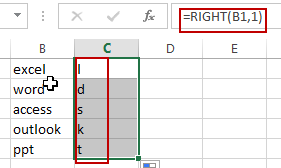#3 you need to keep selecting the cells in helper column, and go to DATA tab, click Sort A to Z command under Sort & Filter group. Then the Sort Warning dialog will open. Select Expand the selection option in the Sort Warning dialog box. Click Sort button.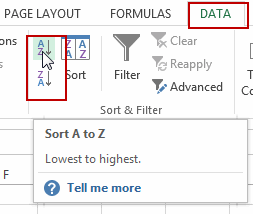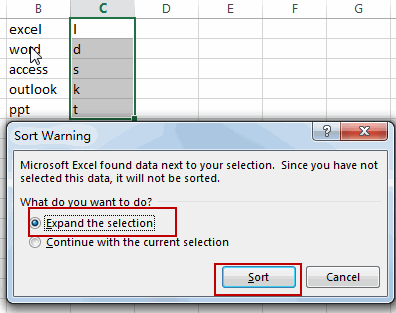#4 you should notice that the range of cells have been sorted. And now you can delete the helper column.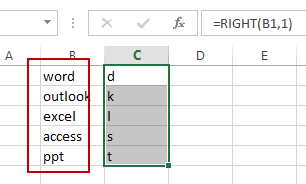## Sorting Data by Last Character with User Defined Function

There is another method to sorting Data by the last character with User Defined Function in Excel. You can try to reverse the text string in Cells, and the last character of original text string will become the first character. So that you can directly sort the cells by the Sort command in the helper column. Here are the steps:

#1 open your excel workbook and then click on “Visual Basic” command under DEVELOPER Tab, or just press “ALT+F11” shortcut.#2 then the “Visual Basic Editor” window will appear.

#3 click “Insert” ->”Module” to create a new module.#4 paste the below VBA code into the code window. Then clicking “Save” button.

```Public Function ReverseString(R As Range)
ReverseString = StrReverse(R.Text)
End Function```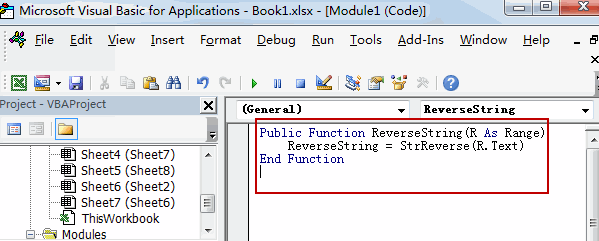#5 back to the current worksheet, then type the following formula in a blank cell, and then press Enter key.

`=ReverseString(B1)`

#6 drag the Fill Handle down to other cells.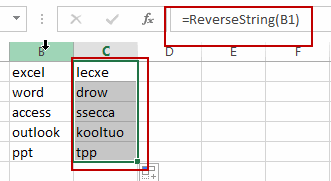#7  keep selecting the cells in helper column, and go to DATA tab, click Sort A to Z command under Sort & Filter group. Then the Sort Warning dialog will open. Select Expand the selection option in the Sort Warning dialog box. Click Sort button.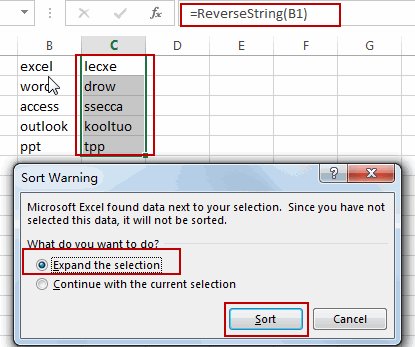#8 you should notice that the range of cells have been sorted. And now you can delete the helper column.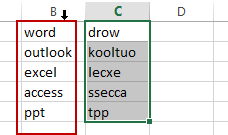### Related Functions

• Excel RIGHT function
The Excel RIGHT function returns a substring (a specified number of the characters) from a text string, starting from the rightmost character.The syntax of the RIGHT function is as below:= RIGHT (text,[num_chars])…

## How to Remove Prefix and Suffix in Given Cells in Excel

This post will guide you how to remove suffix form a range of cells in Excel. How do I remove prefix from text string in cells with a formula in Excel.

Assuming that you have a list of data in range B1:B5, you want to remove the prefix “www.”  or suffix “.com” from the text string in cells. Let’s see the following introduction.

## Remove Prefix of Cells

If you want to remove prefix characters from the text string in range B1:B5, you can use a formula based on the RIGHT function and the LEN function. Like this:

`=RIGHT(B1,LEN(B1)-4)`

Type this formula into a blank cell and press Enter key on your keyboard. And then drag the AutoFill Handle down to cells to apply this formula.

Let’s see how this formula works:

Number 4 is the length of your prefix characters. And the Len function returns the length of the cell B1. The RIGHT function will remove the leftmost four characters (prefix) and return the rest characters of the text string.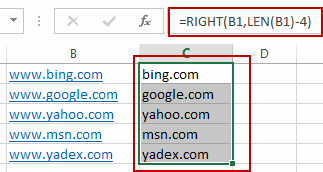## Remove Suffix of Cells

If you want to remove the suffix characters (.com) from the given range of cells in your worksheet, you can use a formula based on the LEFT function and the Len function. Like this:

`=LEFT(B1,LEN(B1)-4)`

Type this formula into a blank cell and press Enter key on your keyboard. And then drag the AutoFill Handle down to cells to apply this formula.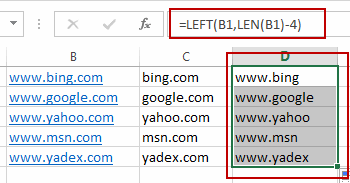## Extract First or Last N Characters

This post will guide you how to extract the first or last n characters from a text string in one cell in Excel. How do I get the first or last N characters from a string using an Excel formula. How to extract first two or last two characters of a cell with a formula in Excel.

## Extract First N Characters

If you want to extract the first n characters of a sting in a cell in Excel, you can use the LEFT function to create a formula to achieve it. For example, you want to extract the first two characters from a text string in Cell B1, just using the following formula:

`=LEFT(B1,2)`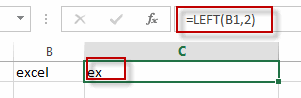Type this formula into a blank cell, and then press Enter key in your keyboard.

The First two characters are extracted in Cell C1.

## Extract Last N Characters

To extract the last N characters in a cell in excel, you need to use the RIGHT function to achieve it. For examples, you want to extract the last two characters from a text string in cell B1, just using the below formula:

`=RIGHT(B1,2)`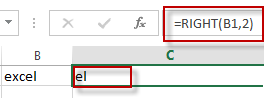Type this formula into a blank cell, and then press Enter key.

The last two characters are extracted in Cell C1.

### Related Functions

• Excel LEFT function
The Excel LEFT function returns a substring (a specified number of the characters) from a text string, starting from the leftmost character.The LEFT function is a build-in function in Microsoft Excel and it is categorized as a Text Function.The syntax of the LEFT function is as below:= LEFT(text,[num_chars])…
• Excel RIGHT function
The Excel RIGHT function returns a substring (a specified number of the characters) from a text string, starting from the rightmost character.The syntax of the RIGHT function is as below:= RIGHT (text,[num_chars])…

## Insert The File Path and Filename into Cell

This post will guide you how to insert the file path and filename into a cell in Excel. Or how to add a file path only in a specified cell with a formula in Excel. Or how to add the current workbook name into a cell in Excel. How do I insert the current worksheet name into a Cell with a formula.

## Insert File Path and Filename into Cell

If you want to insert a file path and filename into a cell in your current worksheet, you can use the CELL function to create a formual. Just like this:

`=CELL("filename")`

Type this formula into a blank cell, and then press Enter key in your keyboard.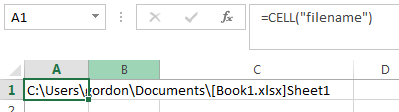This fromula will return the entire path, filename, and tab name of the current worksheet.

If you want to strip out the brackets and the sheet name, you can use the following formula based on the SUBSTITUTE function, the LEFT function, the CELL function and the FIND function:

`=SUBSTITUTE(LEFT(CELL("filename"),FIND("]",CELL("filename"))-1),"[","")`

## Insert Filename Only into Cell

If you only want to insert the workbook name into a cell, you can use a formula based on the MID function, the SEARCH function, and the CELL function. Just like this:

`=MID(CELL("filename"),SEARCH("[",CELL("filename"))+1, SEARCH("]",CELL("filename"))-SEARCH("[",CELL("filename"))-1)`

Type this formula into a blank cell and then press Enter key.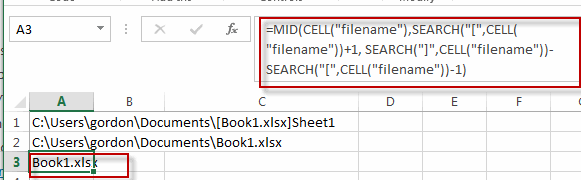You will see this formula will return only the current workbook name in Cell.

## Insert Filepath Only into Cell

If you just want to insert the File Path into a cell, you can create a formula based on the LEFT function, the Cell function,and the FIND function. Just like this:

`=LEFT(CELL("filename",A1),FIND("[",CELL("filename",A1),1)-1)`

Type this formula into a blank cell and then press Enter key to apply this formula.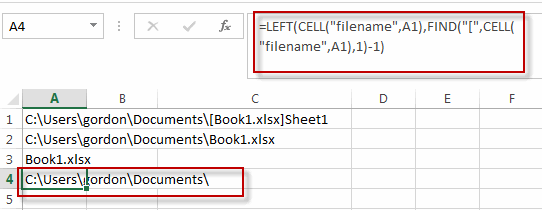This formula will return only the File path of the current workbook.

## Insert Worksheet Name into Cell

If you want to get the worksheet name only and then insert it into one cell, you can create a formula based on the RIGHT function, the LEN function, the CELL function and the FIND function. Just like this:

`=RIGHT(CELL("filename"),LEN(CELL("filename"))-FIND("]",CELL("filename"),1))`

Type this formula into a blank cell and then press Enter key to apply this formula.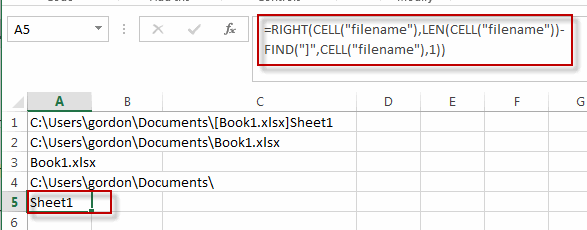This formula will return only the current worksheet name in a cell.

### Related Functions

• Excel Find function
The Excel FIND function returns the position of the first text string (substring) from the first character of the second text string.The FIND function is a build-in function in Microsoft Excel and it is categorized as a Text Function.The syntax of the FIND function is as below:= FIND  (find_text, within_text,[start_num])…
• Excel MID function
The Excel MID function returns a substring from a text string at the position that you specify.The syntax of the MID function is as below:= MID (text, start_num, num_chars)…
• Excel CELL function
The Excel CELL function returns information about the formatting, location, size, or contents of a cell.The syntax of the CELL function is as below:= CELL (info_type,[reference])…
• Excel Substitute function
The Excel SUBSTITUTE function replaces a new text string for an old text string in a text string.The syntax of the SUBSTITUTE function is as below:= SUBSTITUTE  (text, old_text, new_text,[instance_num]) ….
• Excel LEFT function
The Excel LEFT function returns a substring (a specified number of the characters) from a text string, starting from the leftmost character.The LEFT function is a build-in function in Microsoft Excel and it is categorized as a Text Function.The syntax of the LEFT function is as below:= LEFT(text,[num_chars])…
• Excel SEARCH function
The Excel SEARCH function returns the number of the starting location of a substring in a text string.The syntax of the SEARCH function is as below:= SEARCH  (find_text, within_text,[start_num])…
• Excel LEN function
The Excel LEN function returns the length of a text string (the number of characters in a text string).The LEN function is a build-in function in Microsoft Excel and it is categorized as a Text Function.The syntax of the LEN function is as below:= LEN(text)…
• Excel RIGHT function
The Excel RIGHT function returns a substring (a specified number of the characters) from a text string, starting from the rightmost character.The syntax of the RIGHT function is as below:= RIGHT (text,[num_chars])…

## Extract Part of Text String

This post will guide you how to extract part of text string from a cell in Excel. How do I extract a word from a text string in Excel. How to extract a substring from a text string in a Cell in Excel. How to extract a substring from the most left of a text string in Excel. How to extract a word from the most right of a text string in Excel.

Assuming that you have a list of data in the range of cells B1:B5, and you want to get the most left 5 characters from cells, or you want to get the most right 4 characters as a substring from cells, how to achieve it. Let’s see the below detailed steps.

## Extract Word from the Left of a String

To extract word from the left of a text string in a cell in Excel, you can create a formula based on the LEFT function. Like this:

`=LEFT(B1,5)`

Type this formula into a blank cell, then press Enter key in your keyboard. And drag the AutoFill Handle over to other cells to apply this formula.

Let’s see the result: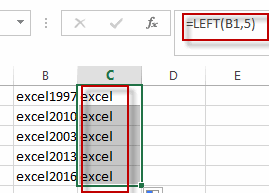You will see that all the most left 5 characters have been extracted into the range C1:C5.

## Extract Word from the right of a String

If you want to extract a substring from the right of a text string in a cell, you can use a formula based on the RIGHT function. Like this:

`=RIGHT(B1,4)`

Type this formula in a blank cell, Press Enter key to apply this formula. Then drag the AutoFill handle over other cells.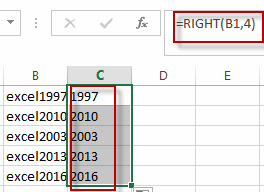## Extract Word from the Mid of a String

If you want to extract a substring from the mid of a text string in a cell, you can use a formula based on the MID function. Like this:

`=MID(B1,6,3)`

This formula will extract a substring from a text string in Cell B1, and the starting position is 6, and the length of substring is 3.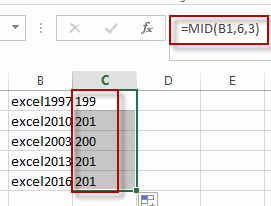### Related Functions

• Excel LEFT function
The Excel LEFT function returns a substring (a specified number of the characters) from a text string, starting from the leftmost character.The LEFT function is a build-in function in Microsoft Excel and it is categorized as a Text Function.The syntax of the LEFT function is as below:= LEFT(text,[num_chars])…t)…
• Excel RIGHT function
The Excel RIGHT function returns a substring (a specified number of the characters) from a text string, starting from the rightmost character.The syntax of the RIGHT function is as below:= RIGHT (text,[num_chars])…
• Excel MID function
The Excel MID function returns a substring from a text string at the position that you specify.The syntax of the MID function is as below:= MID (text, start_num, num_chars)…

## Removing Salutation from Name

This post will guide you how to remove salutations from names in the range of cells in excel. How do I remove the salutation from the names cells with formula in Excel. For example, if you have name salutations and you want to remove those salutations from name strings in excel, how to achieve it. How to split salutation and only names string into separate columns with excel formula.

## Removing Salutation from Name

To remove salutation from names string in excel, you can create a formula based on the RIGHT function, the LEN function and the FIND function.

Assuming that you have a name list in the range of cell B1:B4, and you want to remove all salutations from those cells, you can write down this formula to achieve the result.

`=RIGTH(B1,LEN(B1)-FIND("",B1))`

You just need to type this formula into a blank cell, then press enter key in your keyboard. Then you still need to drag the AutoFill Handler over other cells to apply this formula to remove salutations.

Let’s see the last result: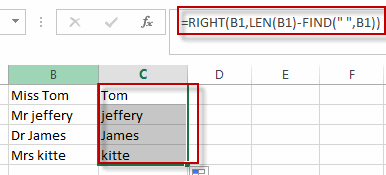Split salutations and name strings into different columns

If you want to split salutations and name string into different columns, you can extract the salutation string firstly, then extract the rest name string again. You need to create the first formula based on the LEFT function and FIND function to extract salutation string. And you still need to use another formula based on the MID function and the FIND function to get the names strings from those cells. Like these two formulas:

```=LEFT(B1,FIND(" ",B1)-1)

=MID(B1,FIND(" ",B1)+1,100)```

Type the first formula into a blank cell to get the salutation strings, then press enter key and drag the AutoFill Handler over other cells.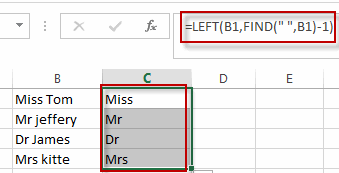Type the second formula into a blank cell in a new column to get the name strings, then press enter key and then drag the AutoFill Handler over other cells to apply this formula to extract names.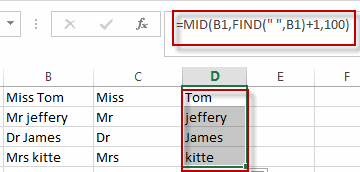### Related Functions

• Excel LEFT function
The Excel LEFT function returns a substring (a specified number of the characters) from a text string, starting from the leftmost character.The LEFT function is a build-in function in Microsoft Excel and it is categorized as a Text Function.The syntax of the LEFT function is as below:= LEFT(text,[num_chars])…t)…
• Excel FIND function
The Excel FIND function returns the position of the first text string (sub string) within another text string.The syntax of the FIND function is as below:= FIND(find_text, within_text,[start_num])…
• Excel RIGHT function
The Excel RIGHT function returns a substring (a specified number of the characters) from a text string, starting from the rightmost character.The syntax of the RIGHT function is as below:= RIGHT (text,[num_chars])…
• Excel MID function
The Excel MID function returns a substring from a text string at the position that you specify.The syntax of the MID function is as below:= MID (text, start_num, num_chars)…
• Excel LEN function
The Excel LEN function returns the length of a text string (the number of characters in a text string).The syntax of the LEN function is as below:= LEN(text)…

This post will guide you how to sort IP address in your worksheet. How do I sort IP address with an excel formula. How to sort IP address with Text To Columns feature in excel.

## Sort IP Address with Formula

Assuming that you have an IP list of data in the range of cells B1:B5, and you want to sort those IP list from low to high, how to achieve the result with excel formula. You need to create a formula based on the Text Function, the LEFT function, the FIND function and the MID function, the RIGHT function. Just like this:

```=TEXT(LEFT(B1,FIND(".",B1,1)-1),"000") & "." & TEXT(MID(B1,FIND(
".",B1,1)+1,FIND(".",B1,FIND(".",B1,1)+1)-FIND(".",B1,1)-1),"000")
& "." & TEXT(MID(B1,FIND(".",B1,FIND(".",B1,1)+1)+1,FIND(".",B1,
FIND(".",B1,FIND(".",B1,1)+1)+1)-FIND(".",B1,FIND(".",B1,1)+1)-1),
"000") & "." & TEXT(RIGHT(B1,LEN(B1)-FIND(".",B1,FIND(".",B1,FIND(
".",B1,1)+1)+1)),"000")```

You will see this formula is quite long, but it is a single one formula and let’s see the detailed steps:

#1 type this formula into the formula box of Cell C1, then press Enter key in your keyboard, and drag the AutoFill Handler over other cells to apply this formula to sort other IP Address.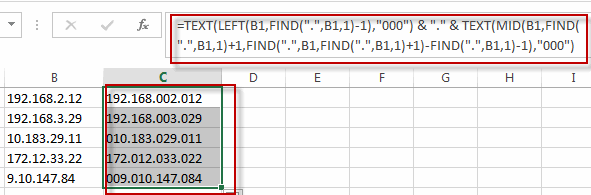#2 keep the newly IP Address selected, and go to DATA tab, click Sort A to Z under Sort&Filter group.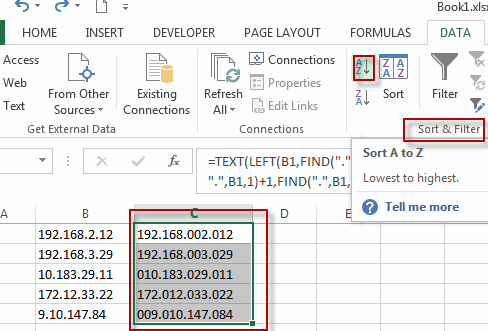#3 select Expand the selection radio button, and then click Sort button.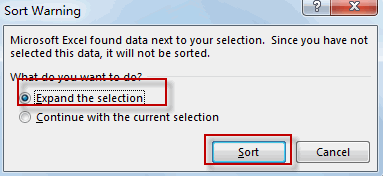#4 you will see the IP addresses have been sorted.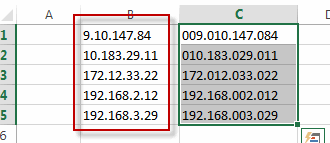## Sort IP Address with Text to Columns

You can also use the Text to Columns feature to sort IP address in excel, You can use this feature to divide the IP Addresses into separate columns and putting each octet in its own column. Before doing those actions, you need to make sure that there are three blank columns next to the right of your IP address. So just do the following steps:

#1 Select the cells that contain IP addresses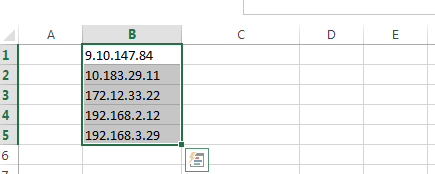#2 go to DATA tab, click Text to Columns command under Data Tools group. The Convert Text to Columns Wizard dialog will appear.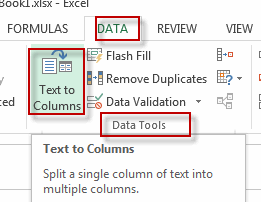#3 Checked Delimited option, and click Next button.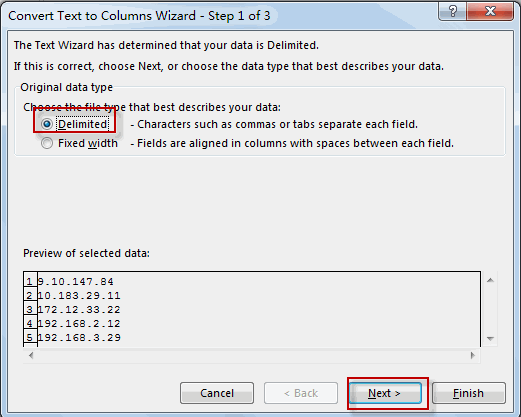#4 select the checkbox next to Other: box and then type . into the text box, and click Next button.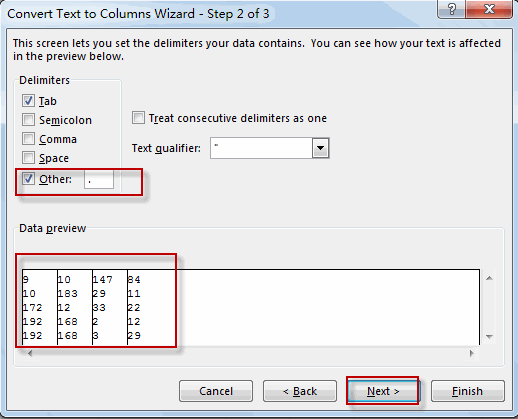#5 select one destination cell to paste the result ( C1). Then click Finsh button.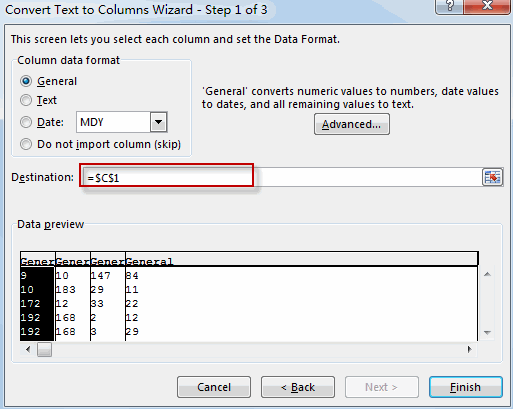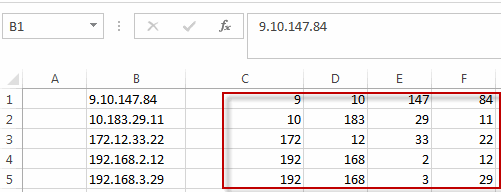#6 select all cells that contain IP addresses.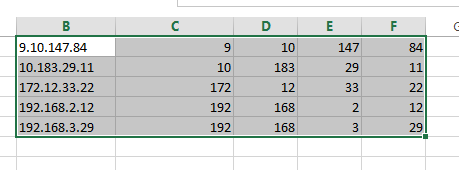#7 click Sort command under Sort&Filter group. The Sort dialog will appear.

#8 clicking Add Level to sort data from column C to F.

#9 click Ok button. You will see that the IP addresses have been sorted.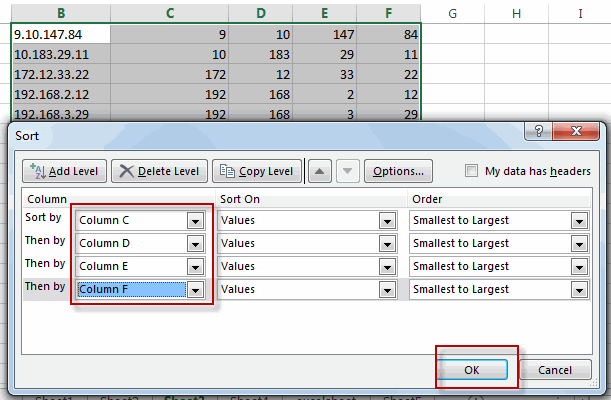### Related Functions

• Excel Text function
The Excel TEXT function converts a numeric value into text string with a specified format. The TEXT function is a build-in function in Microsoft Excel and it is categorized as a Text Function. The syntax of the TEXT function is as below: = TEXT (value, Format code)…
• Excel LEFT function
The Excel LEFT function returns a substring (a specified number of the characters) from a text string, starting from the leftmost character.The LEFT function is a build-in function in Microsoft Excel and it is categorized as a Text Function.The syntax of the LEFT function is as below:= LEFT(text,[num_chars])…t)…
• Excel FIND function
The Excel FIND function returns the position of the first text string (sub string) within another text string.The syntax of the FIND function is as below:= FIND(find_text, within_text,[start_num])…
• Excel RIGHT function
The Excel RIGHT function returns a substring (a specified number of the characters) from a text string, starting from the rightmost character.The syntax of the RIGHT function is as below:= RIGHT (text,[num_chars])…
• Excel MID function
The Excel MID function returns a substring from a text string at the position that you specify.The syntax of the MID function is as below:= MID (text, start_num, num_chars)….

## Highlight Cells That End with Specfic Characters

This post will guide you how to highlight values that end with a specific character or text string in excel. How do I highlight cells that end with a string using the conditional formatting feature in excel.

Assuming that you want to highlight cells that end with the text string “excel” in the range A1:B4, you can refer to the following tow method.

## Highlight Cells that End with a String using Conditional Formatting

If you want to highlight cells that end with a specific text string, you can create a simple formula based on the COUNTIF function to apply conditional formatting. And this formula will check if the selected cells match the given criteria that end with “excel”. Just do the following steps:

#1 select the cells of range that you want to highlight.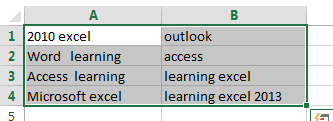#2 go to HOME tab, click Conditional Formatting command under Styles group, then select New Rule… from the drop down list. The New Formatting Rule dialog will appear.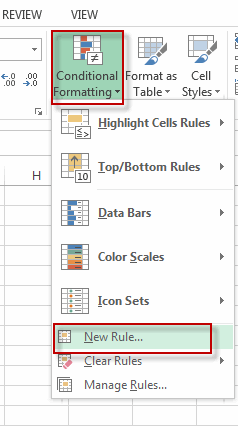#3 select Use a formula to determine which cells to format in the Select a Rule Type section. Then type the formula =COUNTIF(A1,”*excel”) into the text box of  Format values where this formula is true: . Click Format… command, the Format Cells dialog will appear.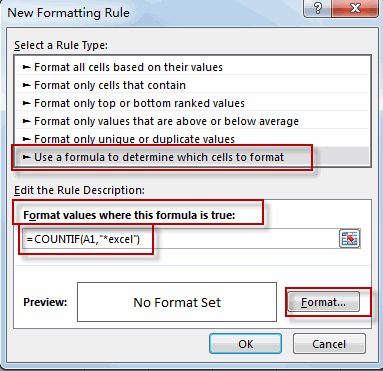#4 click Fill tab, choose one background color as you need. Click OK.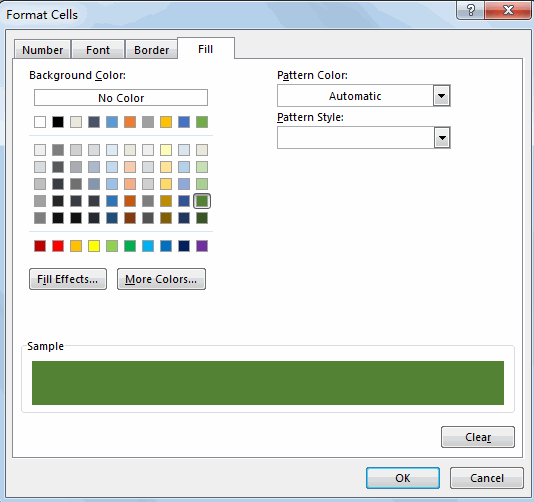#5 click OK. You will see that all cells that end with “excel”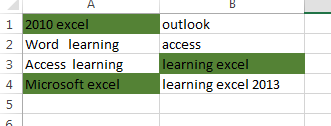Note: the COUNTIF function is not case-sensitive, so if you want to check the cells in case sensitive, you can use a more complicated formula based on the RIGHT function, the EXACT function and the LEN function. Just type the following formula in the above step 3.

=EXACT(RIGHT(A1,LEN(“excel”)),”excel”)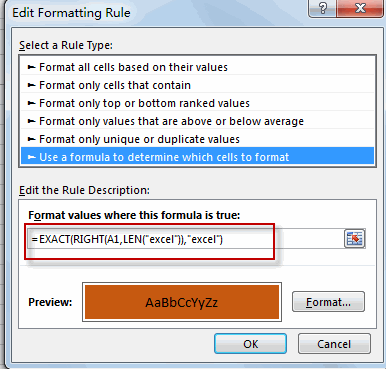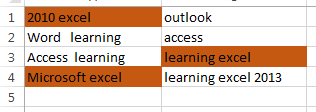The RIGHT function will extract the text from the most right of each cell in the selected cell, and the LEN function will return the length of the specific text string and then passed it into the RIGHT function. The EXACT function will compare the extracted text by RIGHT function to the given text string, if True, then return the TRUE value. Otherwise, it returns FALSE.

### Related Functions

• Excel COUNTIF function
The Excel COUNTIF function will count the number of cells in a range that meet a given criteria. This function can be used to count the different kinds of cells with number, date, text values, blank, non-blanks, or containing specific characters.etc.= COUNTIF (range, criteria)…
• Excel EXACT function
The Excel EXACT function compares if two text strings are the same and returns TRUE if they are the same, Or, it will return FALSE.The syntax of the EXACT function is as below:= EXACT (text1,text2)…
• Excel RIGHT function
The Excel RIGHT function returns a substring (a specified number of the characters) from a text string, starting from the rightmost character.The syntax of the RIGHT function is as below:= RIGHT (text,[num_chars])…
• Excel LEN function
The Excel LEN function returns the length of a text string (the number of characters in a text string).The syntax of the LEN function is as below:= LEN(text)…

## Split Text and Numbers

This post will guide you how to separate text and numbers from one cell in excel. How do I extract numbers from text string with formulas in Excel.

## Split Text and Numbers with Formula

If you want to split text and numbers from one cell into two different cells, you can use a formula based on the FIND function, the LEFT function or the RIGHT function and the MIN function. Just do the following steps:

#1 type the following formula in the formula box of cell C1 to get the Text part from text string in Cell A1.

`=LEFT(A1,MIN(FIND({0,1,2,3,4,5,6,7,8,9},A1&"0123456789"))-1)`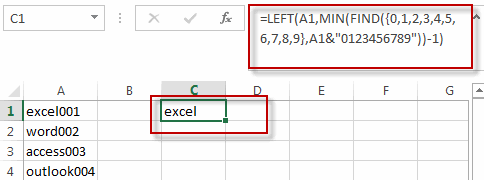#2 type the following formula in the formula box of cell D1 to get the number part from the text string in cell A1.

`=RIGHT(A1,LEN(A1)-MIN(FIND({0,1,2,3,4,5,6,7,8,9},A1&"0123456789"))+1)`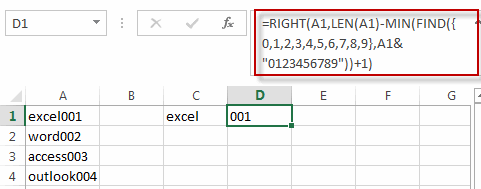3# select the cell C1 and D1, then drag the AutoFill Handle over other cell to apply those formula to split text and numbers.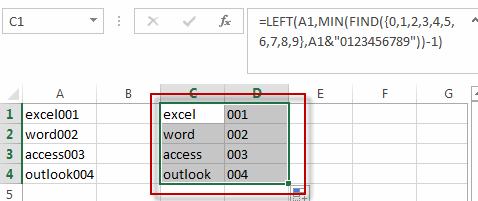## Split Text and Numbers with Flash Fill Feature

You can also use the Flash Fill function to split the text and numbers from the Cell A1, just do the following steps:

#1 type the text part of your first text string into the adjacent blank Cell B1.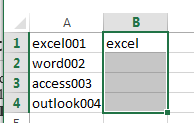#2 select the range B1:B4 where you want to fill the text part, and go to DATA tab, click Flash Fill command under Data Tools group. All text part is filled into the cells B1:B4.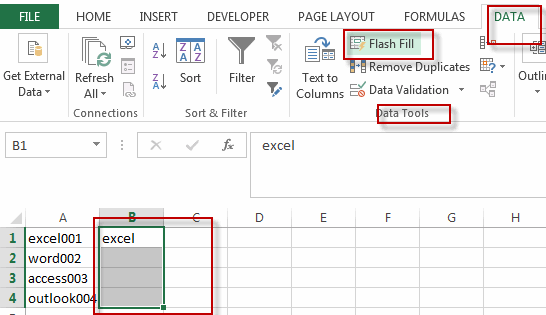#3 enter the number of your first text string into Cell C1.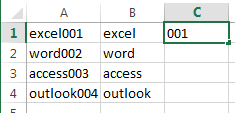#4 select the range D11:D4 where you want to fill the number part, and go to DATA tab, click Flash Fill command. All numbers are filled into the cells D1:D4.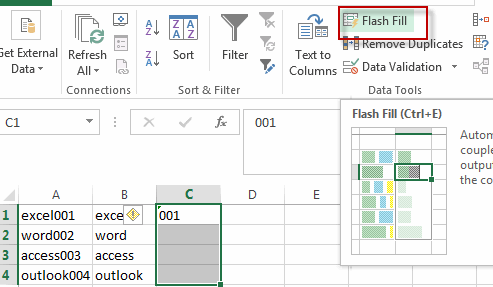#5 Lets see the result.### Related Functions

• Excel LEFT function
The Excel LEFT function returns a substring (a specified number of the characters) from a text string, starting from the leftmost character.The LEFT function is a build-in function in Microsoft Excel and it is categorized as a Text Function.The syntax of the LEFT function is as below:= LEFT(text,[num_chars])…t)…
• Excel FIND function
The Excel FIND function returns the position of the first text string (sub string) within another text string.The syntax of the FIND function is as below:= FIND(find_text, within_text,[start_num])…
• Excel MIN function
The Excel MIN function returns the smallest numeric value from the numbers that you provided. Or returns the smallest value in the array.The MIN function is a build-in function in Microsoft Excel and it is categorized as a Statistical Function.The syntax of the MIN function is as below:= MIN(num1,[num2,…numn])….
• Excel RIGHT function
The Excel RIGHT function returns a substring (a specified number of the characters) from a text string, starting from the rightmost character.The syntax of the RIGHT function is as below:= RIGHT (text,[num_chars])…
• Excel LEN function
The Excel LEN function returns the length of a text string (the number of characters in a text string).The syntax of the LEN function is as below:= LEN(text)…